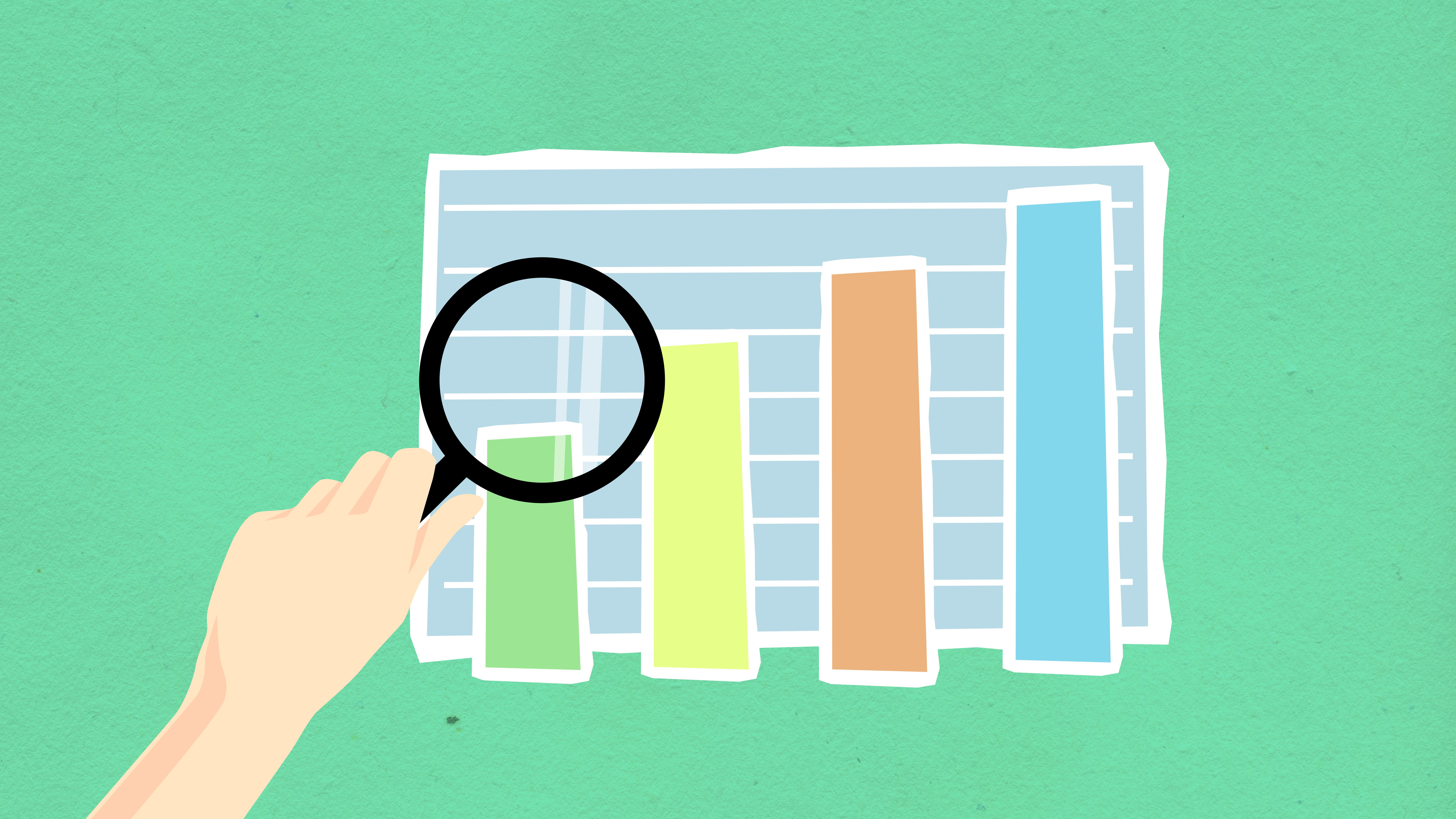# Is graph theory easy to learn?

Is Graph Theory Easy to Learn?

# Is Graph Theory Easy to Learn?## Introduction

Graph theory is an important part of mathematics that deals with the study of graphs, which are a collection of vertices (points) and edges (lines) that connect them. Graphs are used to model all sorts of things, such as social networks, transportation systems, and computer networks. Graph theory is a complex subject, but how easy or hard it is to learn depends on your background knowledge in mathematics. In this article, I’ll help you determine how easy or hard it is to learn graph theory and give you some tips on how to learn it.

## FAQs

### 1. What background knowledge do I need to learn graph theory?

You can learn introductory or algorithmic graph theory with a minimal knowledge of maths. However, for something like extremal graph theory, some analysis and algebra start to become more helpful.

### 2. How should I approach learning graph theory?

There are many resources available online for learning graph theory, such as websites, online courses, and books. It’s important to start with the basics and gradually work your way up to more complex topics. It’s also important to practice solving problems to really solidify your understanding of the subject. Finally, joining a study group or finding a tutor can also help you learn graph theory faster and more efficiently.

### 3. Is graph theory useful in real life?

Graph theory has many applications in real life, such as in computer networking, social networks, and transportation systems. It is used to model and optimize these systems to make them more efficient and cost-effective. Graph theory is also used in many other fields, such as bioinformatics, economics, and physics.

### 4. Are there any prerequisites before learning graph theory?

While you don’t necessarily need any prerequisites before learning graph theory, having a strong foundation in algebra and trigonometry can be helpful. Familiarity with set theory and basic proof techniques can also be helpful in understanding more complex graph theory concepts.

### 5. What are some tips for understanding graph theory concepts better?

One tip for understanding graph theory concepts better is to visualize them. Drawing graphs and looking at them can help you understand the relationships between vertices and edges better. Another tip is to practice solving problems to get a better understanding of the subject. Finally, don’t be afraid to ask for help if you don’t understand something. Joining a study group or finding a tutor can help you learn faster and more efficiently.

## Can Anyone Learn Graph Theory?

Whether or not anyone can learn graph theory depends on their background knowledge in mathematics. A minimal understanding of maths is needed for introductory or algorithmic graph theory, but for more complex topics such as extremal graph theory, some analysis and algebra may be required. However, anyone can learn graph theory with the right resources and approach.

One of the best resources for learning graph theory is the internet. There are many websites and online courses available that can help you learn graph theory at your own pace. Some good websites for learning graph theory include Khan Academy, Brilliant, and Coursera. These websites offer tutorials, videos, and interactive problems to help you learn graph theory.

Another important resource for learning graph theory is books. There are many excellent graph theory books available that cover a wide range of topics, from introductory graph theory to more advanced topics like spectral graph theory. Some good graph theory books include “Introduction to Graph Theory” by Douglas B. West, “Graph Theory” by Reinhard Diestel, and “The Probabilistic Method” by Noga Alon and Joel Spencer.

Finally, joining a study group or finding a tutor can also help you learn graph theory faster and more efficiently. A study group can provide you with support, motivation, and accountability, while a tutor can help you with specific problems and concepts that you may be struggling with.## Is Graph Theory Hard?

Whether or not graph theory is hard depends on your background knowledge in mathematics. If you have a strong foundation in algebra and trigonometry, and are familiar with set theory and basic proof techniques, then you should be able to understand introductory graph theory topics relatively easily. However, if you are not familiar with these concepts, it may take more time and effort to understand graph theory.

Another factor that can make graph theory hard is the level of abstraction involved. Graph theory deals with abstract concepts like vertices and edges, which can be difficult to visualize and understand at first. To overcome this, it’s important to practice drawing graphs and visualizing them to help you understand the concepts better.

Finally, some graph theory topics are more difficult than others. For example, extremal graph theory requires more analysis and algebraic techniques, so it may be more difficult to understand than other topics like planar graphs or bipartite graphs.

See also  Is it better to get a BA or a BS in computer science?## Conclusion

Graph theory is an important part of mathematics that can be easy or hard to learn, depending on your background knowledge. However, with the right resources and approach, anyone can learn graph theory. It’s important to start with the basics and practice solving problems to get a better understanding of the subject. Joining a study group or finding a tutor can also help you learn graph theory faster and more efficiently.

Whether or not graph theory is useful in real life depends on the specific field. However, graph theory has many applications in fields like computer networking, social networks, and transportation systems. It is used to model and optimize these systems to make them more efficient and cost-effective.References: